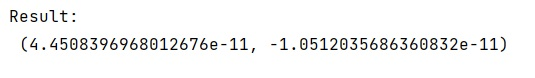# How to solve a pair of nonlinear equations?

Learn, how to solve a pair of nonlinear equations using Python?
Submitted by Pranit Sharma, on January 14, 2023

NumPy is an abbreviated form of Numerical Python. It is used for different types of scientific operations in python. Numpy is a vast library in python which is used for almost every kind of scientific or mathematical operation. It is itself an array which is a collection of various methods and functions for processing the arrays.

## Solving a pair of nonlinear equations

For this purpose, we will use the fsolve() method from optimize module of scipy. This method finds the roots of a function. It returns the roots of the (non-linear) equations defined by func(x) = 0 given a starting estimate.

Syntax:

```scipy.optimize.fsolve(func, x0, args=())
```

Parameter(s):

• funccallable f(x, *args): A function that takes at least one (possibly vector) argument, and returns a value of the same length.
• x0ndarray: The starting estimate for the roots of func(x) = 0.
• argstuple, optional: Any extra arguments to func.

Let us understand with the help of an example,

## Python code to solve a pair of nonlinear equations

```# Importing fsolve method
from scipy.optimize import fsolve

# Import math
import math

# Defining a function for solving equation
# for some values of x and y
def fun(k):
x, y = k
return (x+y**2-4, math.exp(x) + x*y - 3)

# Calling function
x, y =  fsolve(fun, (1, 1))

# Display result
print("Result:\n",fun((x,y)))
```

Output: